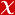## Measurement of the inelastic cross section at 13 TeV

Hadron Structure and QCD: from Low to High Energies, Gatchina, Russia, 27 June - 01 July 2016• Publication Type: Conference Paper / Summary Text
• Doi Number: 10.1103/physrevlett.117.182002
• City: Gatchina
• Country: Russia
• Hacettepe University Affiliated: Yes

#### Abstract

This Letter presents a measurement of the inelastic proton-proton cross section using 60 $\mu$b$^{-1}$ of $pp$ collisions at a center-of-mass energy $\sqrt{s}$ of $13$ TeV with the ATLAS detector at the LHC. Inelastic interactions are selected using rings of plastic scintillators in the forward region ($2.07<|\eta|<3.86$) of the detector. A cross section of $68.1\pm 1.4$ mb is measured in the fiducial region $\xi=M_X^2/s>10^{-6}$, where $M_X$ is the larger invariant mass of the two hadronic systems separated by the largest rapidity gap in the event. In this $\xi$ range the scintillators are highly efficient. For diffractive events this corresponds to cases where at least one proton dissociates to a system with $M_X>13$ GeV. The measured cross section is compared with a range of theoretical predictions. When extrapolated to the full phase space, a cross-section of $78.1 \pm 2.9$ mb is measured, consistent with the inelastic cross section increasing with center-of-mass energy.